# Rational Functions

Rational functions are special functions that you cannot call polynomials, but are obtained by dividing polynomials. In other words, they are the quotients of the polynomial division.

A rational function is of form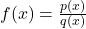where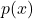and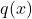are polynomials and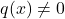. The domain of rational function can be any real numbers except those that makes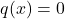.

Example #1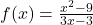is a rational function. The function accept all real number except 1. The value 1 makes the function invalid. Hence, we can write domain of the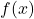as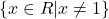.

Example #2

Find the domain of rational function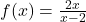.

Solution:

The rational function accepts all values, except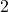, which we can write in interval notation also,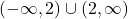.

### The Reciprocal Function

The simplest of rational function is the reciprocal function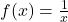. The function accepts all real values except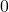.

Let us plot the graph of this rational function for following values.

As the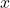value approachesfrom left, the value ofbecomes smaller and smaller boundlessly to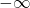. This can be shown with the arrow notation as follows.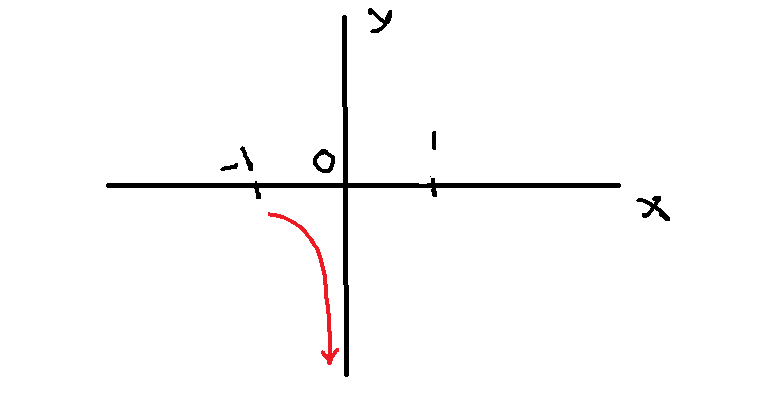Figure 1 – As x approaches 0 from left, the f(x) decreases boundlessly to negative infinity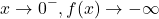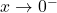,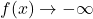What happens whencomes closer tofrom right side.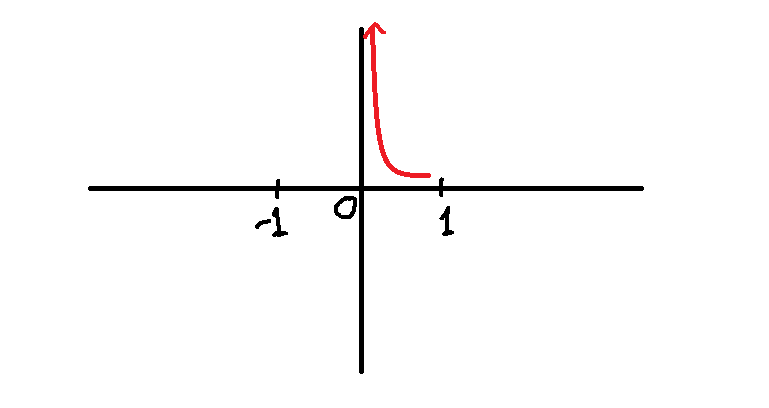Figure 2 – As x decreases and comes closer to 0, the f(x) becomes larger and moves towards positive infinity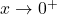,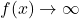.

As thevalue approaches 0 from right , the value ofincreases boundlessly to positive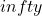. This is shown above with arrow notation.

What happens when the value ofmoves away from, that is,increases or decreases boundlessly ?

When thevalue increases or decreases boundlessly, then theapproaches, but not touching.

This is shown in arrow notation below.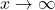,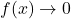and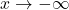,.

### Vertical Asymptotes

There are several rational functions, out of which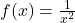is an interesting one. The graph of this function is reflected across the y-axis.

We can describe the end behavior of this graph in the following manner.

The line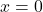is called the vertical asymptote of the graph. A rational function can have

1. one vertical asymptote
2. many vertical asymptotes
3. or no vertical asymptotes

The end behavior of rational function around vertical asymptote are:

Figure 7 – As x approaches a, f(x) increases boundless

As the value ofapproaches the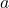, theincreases or decreases without bound.

This increase or decrease in end behavior is useful in study of calculus. We can describe how the value ofand the function changes using limits.

Themoves closer tofrom left or right, the end behavior changes is shown in limits below.

### How To Locate The Vertical Asymptotes ?

If the rational function have vertical asymptotes, then it can be found easily. We know thatwhereandare two polynomials. There are two conditions to find the vertical asymptotes:

1. The polynomialsandhave no common factors, if they have common factor must be eliminated.
2. The valuemust be zero of the polynomial, that is, denominator. Ifis zero then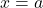is the vertical asymptotes.

You can understand this with the help of an example.

Example #3

Find the vertical asymptotes of the rational function: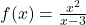Solution:

The given equationdoes not have any common factors, therefore, meet the first condition. The denominator accepts all real numbers except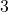which makes it.

Therefore,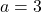is the vertical asymptote in the graph of rational function.

Example #4

Find the vertical asymptote of the rational function: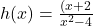.

Solution:

In the given equation,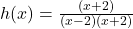has a common factor. We reduce the common factor and the equation becomes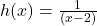.

The function accepts all real values,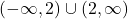except. Therefore,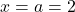is the vertical asymptotes.

Example #5

Find the vertical asymptotes for the rational function: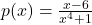.

Solution:

The function has no common factor, but there is novalue for which the denominator is. Therefore, the function does not have a vertical asymptote.

In some cases, the denominator is shows that it has a zero, but after reducing the common factors, the resultant expression has a totally new vertical asymptote.

Example #6

Find the vertical asymptote for the equation: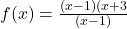.

Solution:

At first we see that the equation has a zero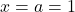, but when the equation is reduced after reducing the common factors, we get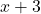and the vertical asymptote is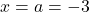.

### Horizontal Asymptotes

The equationrepresents “vertical asymptote“, similarly,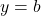represents the “horizontal asymptotes“. There may be several vertical asymptotes, but there is only one horizontal asymptote.

The function, asincreases or decreases without boundor, theapproaches, which is. So, we can say that the horizontal asymptote is that which is defined as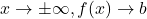.